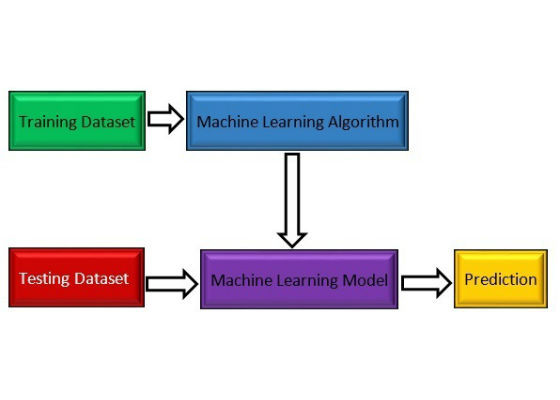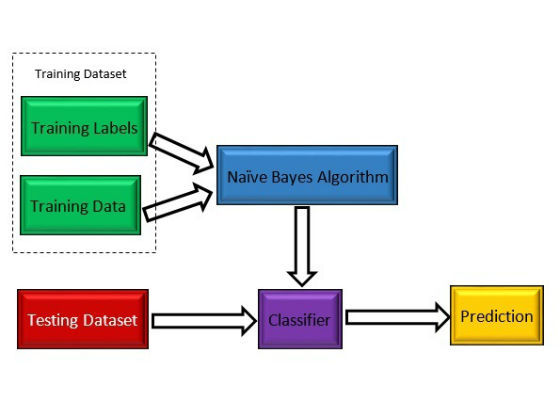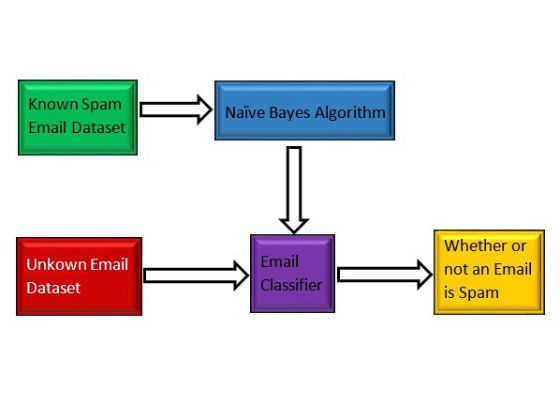Using Intel Data Analytics Acceleration Library to Improve the Performance of Naïve Bayes Algorithm in Python

By Promotion | Published on Dec 28 2016Introduction

How is Netflix able to recommend videos for customers to view? How does Amazon know which products to suggest to potential buyers? How does Microsoft Outlook* categorize an email as spam?

How do they do it? Netflix is able to recommend videos to customers from past viewing experience. Amazon uses data from shopper viewing and buying histories to suggests products that shoppers might likely to buy. Microsoft analyzes a huge amount of emails to determine whether or not an email is junk or spam.

It seems like Netflix, Amazon, and Microsoft have done some kind of analysis on the historical databases of some kind to come up with decisions to assist their customers with their needs. With the availability of a huge amount of data (video, audio, and text) on social media and on the Internet, there needs to be an efficient way to handle these big sets of data with minimum human intervention in order to gain more insight into how people think, behave, and interact with society and our environment.

This has led to what we call machine learning .

This article discusses machine learning and describes a machine learning method/algorithm called Naïve Bayes (NB) . It also describes how to use Intel® Data Analytics Acceleration Library (Intel® DAAL)  to improve the performance of an NB algorithm.

What is Machine Learning?

Machine learning (ML) is a data analysis method that is used to create an analytical model base on a set of data. That analytic model has the ability to learn without being explicitly programmed as new data is being fed to it. ML has been around for quite some time, but only recently has it proved to be useful due to the following:

• The growing volumes and varieties of available data from social media and on the Internet are increasingly available.
• Computer systems are getting more powerful.
• Data storages are getting larger and cheaper.

The two most common types of ML are supervised learning  and unsupervised learning .
With supervised learning, algorithms are trained using a set of input data and a set of labels (known results.) Each time algorithms learn by comparing the results of the input data with the label and adjust the machine learning model. Classification is considered supervised learning.

Unlike supervised learning, with unsupervised learning algorithms have no labels to learn from. Instead, they have to look through input data and detect patterns on their own. For example, to categorize which region in the world a person belongs to, the algorithms have to look at the population data, identify races, religions, languages, and so on.Figure 1: Simplified machine learning diagram.

Figure 1 shows a simplified diagram of how ML works. First, an ML algorithm gets trained using a training data set and creates an ML model. That model processes the testing data set and, finally, the result will be predicted.

The next section discusses a type of supervised learning algorithm, the Naïve Bayes algorithm.

Naïve Bayes Algorithm

The Naive Bayes (NB) algorithm is a classification method based on Bayes’ theorem  with the assumption that all features are independent of each other.

Bayes’ theorem is represented by the following equation:Where X and Y are features

• P(Y|X) is the probability of Y given X.
• P(X|Y) is the probability of X given Y.
• P(Y) is the prior probability of Y.
• P(X) is the prior probability of X.

From , the NB equation can be written as follows:Where X = (x1, x2,… xn) represents a vector of n features.

The NB algorithm is commonly used in detecting email sorting, document categorization, email spam detection, and so on.
The diagram in Figure 2 shows how the NB algorithm works:Figure 2: Machine learning diagram with Naïve Bayes algorithm.

Figure 2 shows that the training data set consists of two sets: the training labels and the training data. The training labels are the correct outputs of the training data. These two sets are needed to build the classifier. After that, the testing data set will be fed to the classifier to be evaluated.Figure 3: Email spam detection using Naïve Bayes algorithm.

Figure 3 shows the flow diagram of the NB algorithm detecting email spam. The known spam email goes through the NB algorithm to build the email classifier. After that, unknown emails go through the email classifier to check whether or not an email is spam.

Applications of NB

The following are some NB applications:

• Taking advantage of its speed to make predictions in real time
• Multiclass/multinomial classification, such as classifying many types of flowers
• Detecting spam emails
• Classifying text

The following are some of the advantages and disadvantages of NB.

• Training the model can be done quickly.
• NB does well with multi-class prediction.

• If the data has no label in the training data set, NB cannot make a prediction.
• NB does not work well with large data sets.
• Features/events are not always completely independent.

It takes a considerable amount of time to train the model with a large data set. It can take weeks and even months to train a particular model. To help optimize this training step, Intel developed Intel DAAL.

Intel® Data Analytics Acceleration Library

Intel DAAL is a library that consists of many basic building blocks that are optimized for data analytics and machine learning. These basic building blocks are highly optimized for the latest features of the latest Intel® processors. More about Intel DAAL can be found at . Multinomial Naïve Bayes classifier is one of the classification algorithms that DAAL provides. In this article, we use PyDAAL, the Python API of DAAL, to build a basic Naïve Bayes classifier. To install PyDAAL, follow instructions in .

Using the NB Method in the Intel Data Analytics Acceleration Library

This section shows how to invoke the multinomial NB algorithm  in Python  using Intel DAAL.
Do the following steps to invoke the NB algorithm from Intel DAAL:

1) Import the necessary packages using the commands from and import
a) Import Numpy using the command:
import numpy as np
b) Import the Intel DAAL numeric table using the following command:
from daal.data_management import HomogenNumericTable
c) Import the NB algorithm using the following commands:
from daal.algorithms.multinomial_naive_bayes import prediction, training
from daal.algorithms import classifier
2) Create a function to split the input data set into the training data, label, and test data.

Basically, split the input data set array into two arrays. For example, for a dataset with 100 rows, split it into 80/20: 80 percent of the data for training and 20 percent for testing. The training data will contain the first 80 lines of the array input dataset, and the testing data will contain the remaining 20 lines of the input dataset.

3) Restructure the training and testing dataset so it can be read by Intel DAAL.
Use the commands to reformat the data as followed (We treat ‘trainLabels’ and ‘testLabels’ as n-by-1 tables, where n is the number of lines in the corresponding datasets):

trainInput = HomogenNumericTable(trainingData)
trainLabels = HomogenNumericTable(trainGroundTruth.reshape(trainGroundTruth.shape,1))
testInput = HomogenNumericTable(testingData)
testLabels = HomogenNumericTable(testGroundTruth.reshape(testGroundTruth.shape,1))
Where:
trainInput: Training data has been reformatted to Intel DAAL numeric tables.
trainLabels: Training labels has been reformatted to Intel DAAL numeric tables .
testInput: Testing data has been reformatted to Intel DAAL numeric tables.
testLabels: Testing labels has been reformatted to Intel DAAL numeric table
4) Create a function to train the model
a) First create an algorithm object to train the model using the following command:

algorithm = training.Batch_Float64DefaultDense(nClasses)
b) Pass the training data and label to the algorithm using the following commands:
algorithm.input.set(classifier.training.data, trainInput)
algorithm.input.set(classifier.training.labels, trainLabels)
where:
algorithm: The algorithm object as defined in step a above.
trainInput: Training data.
trainLabels: Training labels.

c) Train the model using the following command:
Model = algorithm.compute()
where:

algorithm: The algorithm object as defined in step a above.

5) Create a function to test the model.

a) First create an algorithm object to test/predict the model using the following command:
algorithm = prediction.Batch_Float64DefaultDense(nClasses)
b) Pass the testing data and the train model to the model using the following commands:
algorithm.input.setTable(classifier.prediction.data, testInput)
algorithm.input.setModel(classifier.prediction.model, model.get(classifier.training.model))
where:

algorithm: The algorithm object as defined in step a above.
testInput: Testing data.
model: Name of the model object

c) Test/predict the model using the following command:
Prediction = algorithm.compute()

where:
algorithm: The algorithm object as defined in step a above.
prediction: Prediction result that contains predicted labels for test data.

For more such intel Machine Learning and tools from Intel, please visit the Intel® Machine Learning Code

Source: https://software.intel.com/en-us/articles/using-intel-data-analytics-acceleration-library-improve-performance-of-na-ve-bayesPopular Mobile Phones

View All

Digit caters to the largest community of tech buyers, users and enthusiasts in India. The all new Digit in continues the legacy of Thinkdigit.com as one of the largest portals in India committed to technology users and buyers. Digit is also one of the most trusted names when it comes to technology reviews and buying advice and is home to the Digit Test Lab, India's most proficient center for testing and reviewing technology products.

We are about leadership-the 9.9 kind! Building a leading media company out of India.And,grooming new leaders for this promising industry.